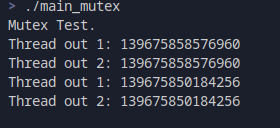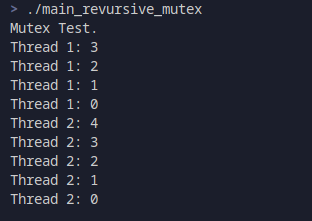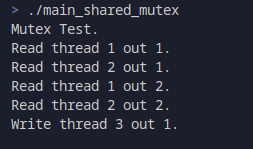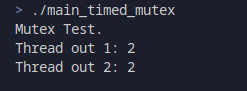## 最基本的锁 std::mutex

### 使用

`std::mutex`是最基本的锁，也是最常见的锁。它提供了最基本的多线程编程同步方法。

``````using namespace std::chrono_literals;

std::mutex g_mutex;

g_mutex.lock();
g_mutex.unlock();
}

int main() {
std::cout << "Mutex Test." << std::endl;
return 0;
}
``````### 方法和属性

• `lock()` 为对象加锁，如果已经被锁了，则阻塞线程；
• `try_lock()` 尝试加锁，如果已经被加锁，则返回false，否则将对其进行加锁并返回true；
• `unlock()` 为对象解锁，通常和加锁（`lock()``try_lock()`）成对出现；
• `native_handle()` 返回锁的POSIX标准对象。

## 递归锁 std::recursive_mutex

`std::recursive_mutex`是一个递归锁，方法和使用都和`std::mutex`类似。唯一的不同是，`std::mutex`在同一时间，只允许加锁一次，而`std::revursive_mutex`允许同一线程下进行多次加锁。如：

``````// 定义递归锁
std::recursive_mutex g_mutex;

// 线程函数
g_mutex.lock();
std::cout << "Thread " << thread_id << ": " << time << std::endl;
g_mutex.unlock();
}

// 初始化线程
``````## 共享锁 std::shared_mutex (C++17)

`std::shared_mutex`在C++14已经存在了，但是在C++14中的`std::shared_mutex`是带timing的版本的读写锁（也就是说，C++14中的`std::shared_mutex`等于C++17中的`std::shared_timed_mutex`）。读写锁有两种加锁的方式，一种是`shared_lock()`，另一种`lock()``shared_lock`是读模式，而`lock`是写模式。读写锁允许多个读加锁，而写加锁和其他所有加锁互斥。即同一时间下：

• 允许多个线程同时读；
• 只允许一个线程写；
• 写的时候不允许读，读的时候不允许写。

``````// 共享锁
std::shared_mutex g_mutex;

// 读线程 1
// 第一个获取读权限
g_mutex.lock_shared();
// 睡眠2s,等待读线程2，获取读权限，确认可以多个线程进行读加锁
// 解锁读
g_mutex.unlock_shared();
}

// 睡眠500ms，确保读线程1先获取锁
g_mutex.lock_shared();
g_mutex.unlock_shared();
}

// 确保读线程先获得锁，确认读写互斥
g_mutex.lock();
std::cout << "Write thread " << thread_id << " out 1."  << std::endl;
g_mutex.unlock();
}

``````## 带超时的锁

``````void thread_func(int thread_id) {
if (!g_mutex.try_lock_for(0.5s)) return;
g_mutex.unlock();
g_mutex.native_handle();
}
``````Tags: